### How to interpret fold-changes when the sample purity is not 100%

If your sample purity is less than 100%, it is necessary to take that into account when interpreting the fold-change values. Given a sample purity of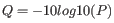%, and an amplification with an observed fold-change of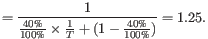, the following formula gives the actual fold-change that would be seen if the sample were 100% pure:

 fold-change in 100% pure sample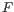(29.23)

For example, if the sample purity is 40%, and you have observed an amplification with a fold-change of 3, then the fold-change in the 100% pure sample would have been:

 fold-change in 100% pure sample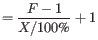(29.24)

For a deletion the formula for converting an observed (absolute) fold-change to the actual (absolute) fold change is:

 fold-change in 100% pure sample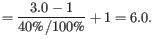(29.25)

For example, if the sample purity is 40%, and you have a deletion with an absolute fold-change of 1.25, then the absolute fold-change in the 100% pure sample would have been:

 fold-change in 100% pure sample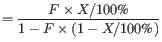(29.26)

Figures 29.27 and 29.28 shows the 'true' fold changes for different observed fold-changes at different sample purities.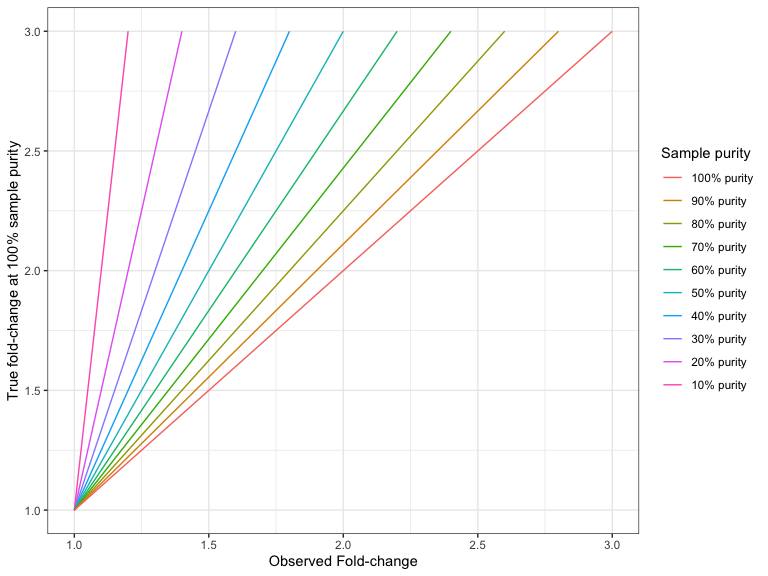Figure 29.27: The true amplification fold-change in the 100% pure sample, for different observed fold-changes, as a function of sample purity.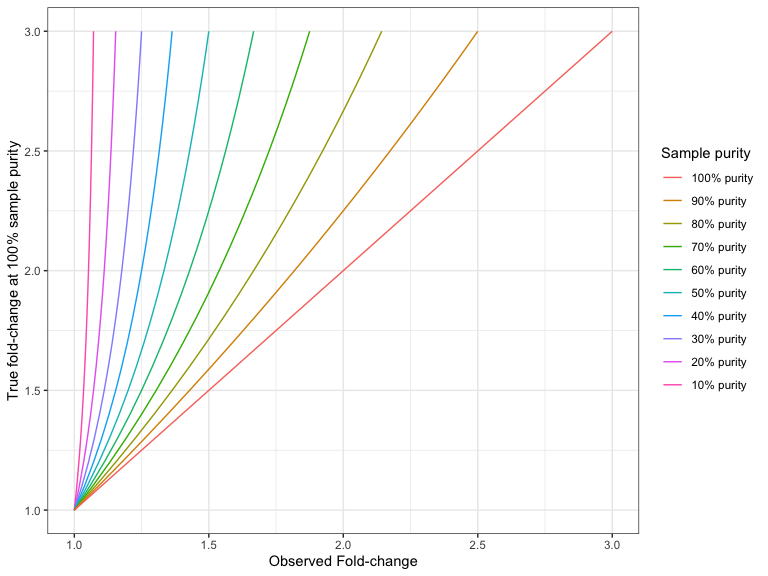Figure 29.28: The true deletion fold-change in the 100% pure sample, for different observed fold-changes, as a function of sample purity.